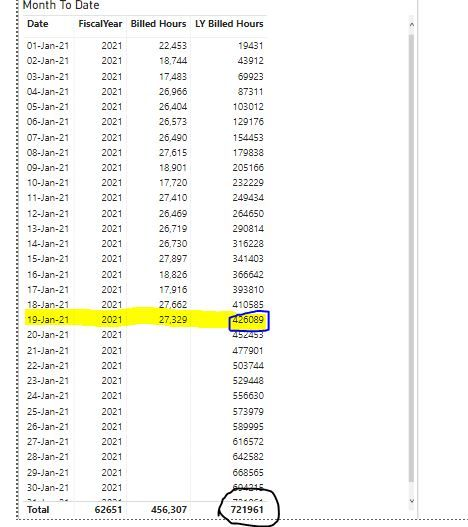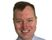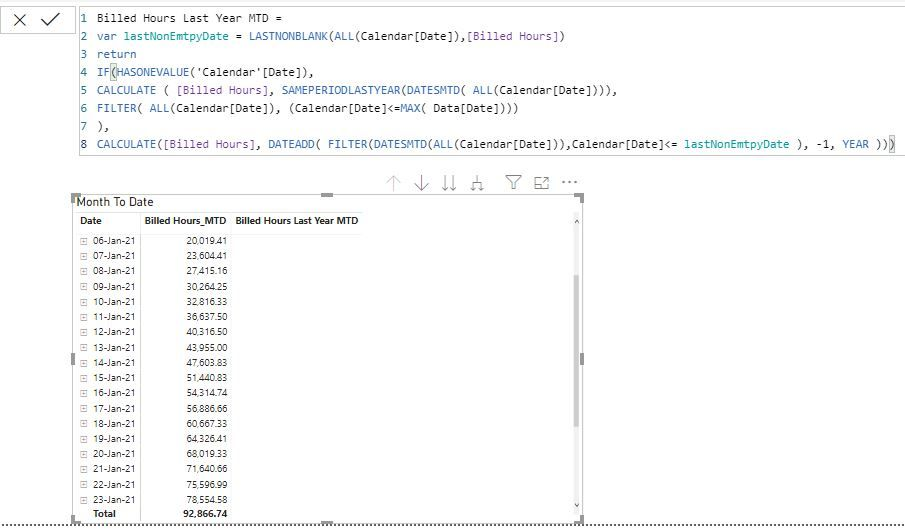cancel
Showing results for
Did you mean:Helper I

## MTD and YTD calculation

@ Power BI users,

When I try to use SAMEPERIODLASTYEAR to get MTD of previous year, it returns entire months billed hours sum, rather I was looking for only till current year date sum as in screenshot below. Also when i try to use Dateadd function Billed Hours previous Year, it gives me the entire year total

Example- I want to view this year MTD ie from Jan 1-Jan 19, 2021 Vs Jan1-Jan 19, 2020 (Screenshot below). Currently i am getting the entire month total highlighted in black but i want to get total till Jan 19, 2020 highlighted in blue.

Following are the measures i used

Billed Hours current Year = Sum(FACT_Monthly_Sales_By_business_Line[Duration Billed])
Billed Hours Last_Year_MTD = CALCULATE ( [Billed Hours], SAMEPERIODLASTYEAR(DATESMTD( 'Calendar'[Date])))
Billed Hours Last_Year_YTD  =  CALCULATE([Billed Hours], DATEADD('Calendar'[Date], -1, YEAR))I tried using this link too but was unable to get the expected result.
1 ACCEPTED SOLUTIONSuper User
``````Billed Hours Last Year MTD =
var lastNonEmtpyDate = LASTNONBLANK(ALL(Calendar[Date]),[Billed Hours])
return
IF(HASONEVALUE('Calendar'[Date]),
CALCULATE ( [Billed Hours], SAMEPERIODLASTYEAR(DATESMTD( (Calendar[Date]))),
FILTER( ALL(Calendar[Date]), Calendar[Date]<=MAX( 'Billed Hours MTD dax'[Date]))
),
CALCULATE([Billed Hours], DATEADD( FILTER(DATESMTD((Calendar[Date])),Calendar[Date]<= lastNonEmtpyDate ), -1, YEAR )))``````

Proud to be a Super User!

13 REPLIES 13Super User

If I understand your issue correctly you want the calculation to stop at the last date that has data. So something like the following might do the trick:

Billed Hours Last Year MTD =
var lastNonEmtpyDate = LASTNONBLANK(ALL(Calendar[Date]),[Bill Hours])
return CALCULATE( [Billed Hours], DATEADD( FILTER(DATESMTD(Calendar[Date]), Calendar[Date] <= lastNonEmtpyDate ), -1, YEAR ))Super User

You need to add a filter statement at the end of your measure to limit dates

your meausre will be look like this.

Billed Hours Last_Year_MTD = CALCULATE ( [Billed Hours], SAMEPERIODLASTYEAR(DATESMTD( 'Calendar'[Date])),FILTER(ALL( 'Calendar'[Date]), 'Calendar'[Date]<=MAX( FACT_Monthly_Sales_By_business_Line[Date]))
)

Proud to be a Super User!Helper I

Hi FarhanAhmed,

The measure did solve my problem to some extent but not completely. If you refere the screenshot below , you can see that though the measure restricts it to maxdate but the total for the previous year still shows the total for the entire month (721961) and not the current date for last year (426089). Screenshot below for reference.Super User

I think the solution suggest by @d_gosbell  will work and you may want to do some tweaking by adding HASONEVALUE in the code to not return values for future dates.

You may give it a try as well.

``````Billed Hours Last Year MTD =
var lastNonEmtpyDate = LASTNONBLANK(ALL(ALL(Calendar[Date]),[Bill Hours])
return
IF(HASONEVALUE(ALL(Calendar[Date]),

CALCULATE ( [Bill Hours], SAMEPERIODLASTYEAR(DATESMTD( ALL(Calendar[Date])),FILTER(ALL( ALL(Calendar[Date]), ALL(Calendar[Date]<=MAX( Query1[Date]))
)
,
CALCULATE( [Bill Hours], DATEADD( FILTER(DATESMTD(ALL(Calendar[Date]),ALL(Calendar[Date] <= lastNonEmtpyDate ), -1, YEAR )))``````

Proud to be a Super User!Helper I

Hi FarhanAhmed,

I am facing some issue with the the above formula as in screenshot below. Also i have attached my Pbix file in the link below for reference.It would be great if you could review the file and let me know where i am missingSuper User

Sorry can't open pbix right now

But There seems to be 2 ALL in LASTNONBLANK with Calendar date which is causing this error

Also make sure that brackets are closed properly.

Proud to be a Super User!Helper I

Hi FarhanAhmed,

I guess this time i tried rectifying and closing the brackets but still unable to get there. Any leads would be appreciated.Super User

Seems like first calculate missing 1 close bracket and 2nd one has 1 extra bracket

Proud to be a Super User!Helper I

Thanks for the quick correction Farhan. The Dax works but somehow i get no value populated in the visual as below 😑.. Any insights?Super User
``````Billed Hours Last Year MTD =
var lastNonEmtpyDate = LASTNONBLANK(ALL(Calendar[Date]),[Billed Hours])
return
IF(HASONEVALUE('Calendar'[Date]),
CALCULATE ( [Billed Hours], SAMEPERIODLASTYEAR(DATESMTD( (Calendar[Date]))),
FILTER( ALL(Calendar[Date]), Calendar[Date]<=MAX( 'Billed Hours MTD dax'[Date]))
),
CALCULATE([Billed Hours], DATEADD( FILTER(DATESMTD((Calendar[Date])),Calendar[Date]<= lastNonEmtpyDate ), -1, YEAR )))``````

Proud to be a Super User!Helper I

Hi FarhanAhmed,

Thankyou for the Magic!! Saved my day!!Helper I

Hi FarhanAhmed,

Can you email your mail id to send the Pbix?Super User

You are nearly there. In addition to SAMEPERIODLASTYEAR you also need a filter from your dates table that includes all dates that are older than or equal to "Today minus one year". Depending on your dataset refresh frequency the easiest way to implement that is as a calculated column that gets updated during refresh.